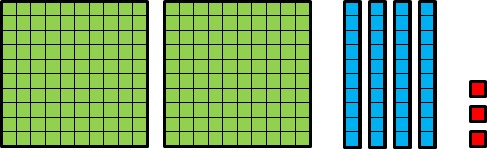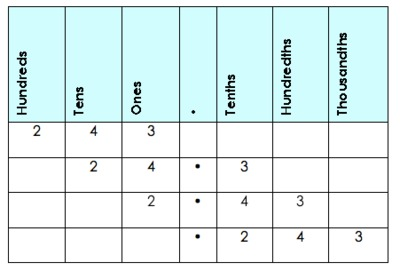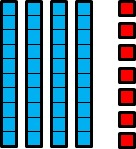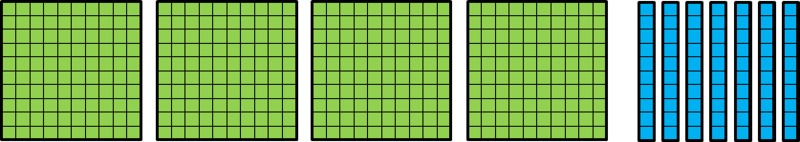# Which number is it?

Alignments to Content Standards: 5.NBT.A.1

Netta drew a picture on graph paper:She said,

In my picture, a big square represents 1. Since ten rectangles make a big square, a rectangle represents 0.1. Since 100 little squares make a big square, a little square represents 0.01. So this picture represents 2.43.
1. Is Netta Correct?
2. Manny said,
I drew the same picture, but in my picture, a little square represents 1, so this picture represents 243.
3. Name some other numbers that this picture could represent. For each of these numbers, what does a little square represent? What does a rectangle represent? What does a big square represent? Explain.
4. Draw a picture to represent 0.047.

## IM Commentary

The purpose of this task is to help students understand the fact that the value of a digit in one place is ten times the value of the same digit in the place to the right. Given that students will likely only have used base-ten blocks with the unit cube representing one, this task should not be introduced all at once if students are not familiar with unitizing (changing the definition of the whole and assigning value based on the relationship to the unit). The first part to this task alone could be expanded to a full lesson on how one arrangement of base ten blocks could represent more than one number depending on whether we see the large square as the whole or the small square as the whole. These two representations tend to work well for students making that jump because they can directly see that one small square is one hundredth of the large square. The other representations are more abstract for students and may be introduced once students are comfortable defining different blocks to be the unit.

A place value organizer can be an effective tool to summarize student work. Students should be encouraged to grapple with this task before seeing the place value organizer. After the students struggle and create different numbers, the place value organizer can be used to record different student ideas about "numbers that might work." Given three or more public student examples, ask students to create a generalization about numbers that will work.## Solutions

Solution: 1

1. If a little square represents 0.1, then a rectangle represents 1 because ten tenths make 1. A big square represents 10 since ten ones makes 10. So this picture could represent 24.3.

2. If a little square represents 0.001, then the picture below represents 0.047:Solution: 2

1. If a little square represents 0.001, then a rectangle represents 0.01 because ten thousandths make one hundredth. A big square represents 0.1 since ten hundredths make a tenth. So this picture could represent 0.243.

2. If a rectangle represents 0.001, then the picture below represents 0.047: